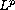Electron. J. Diff. Eqns., Vol. 2007(2007), No. 37, pp. 1-21.

### On a class of nonlinear variational inequalities: High concentration of the graph of weak solution via its fractional dimension and Minkowski content Luka Korkut, Mervan Pasic

Abstract:
Weak continuous bounded solutions of a class of nonlinear variational inequalities associated to one-dimensional p-Laplacian are studied. It is shown that a kind of boundary behaviour of nonlinearity in the main problem produces a kind of high boundary concentration of the graph of solutions. It is verified by calculating lower bounds for the upper Minkowski-Bouligand dimension and Minkowski content of the graph of each solution and its derivative. Finally, the order of growth for singular behaviour of thenorm of derivative of solutions is given.

Submitted November 19, 2006. Published March 1, 2007.
Math Subject Classifications: 35J85, 34B15, 28A75.
Key Words: Double obstacles; nonlinear p-Laplacian; graph; fractional dimension; Minkowski content; singularity of derivative.

Show me the PDF file (347K), TEX file, and other files for this article.

 Luka Korkut Department of mathematics Faculty of Electrical Engineering and Computing University of Zagreb Unska 3, 10000 Zagreb, Croatia e-mail: luka.korkut@fer.hr Mervan Pasic Department of mathematics Faculty of Electrical Engineering and Computing University of Zagreb Unska 3, 10000 Zagreb, Croatia e-mail: mervan.pasic@fer.hr